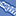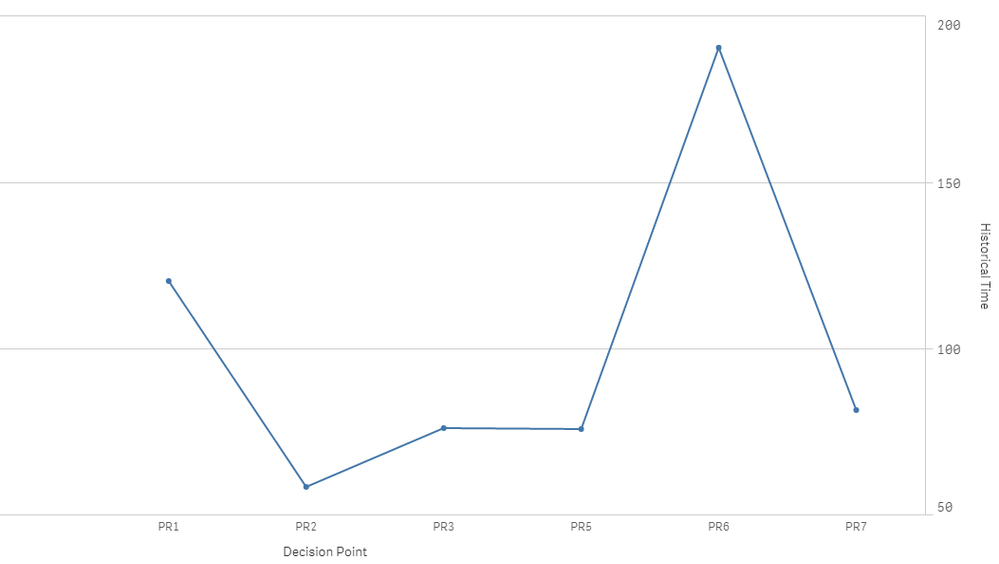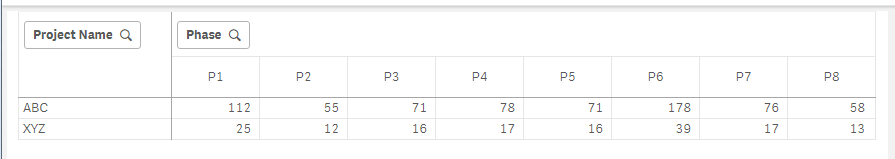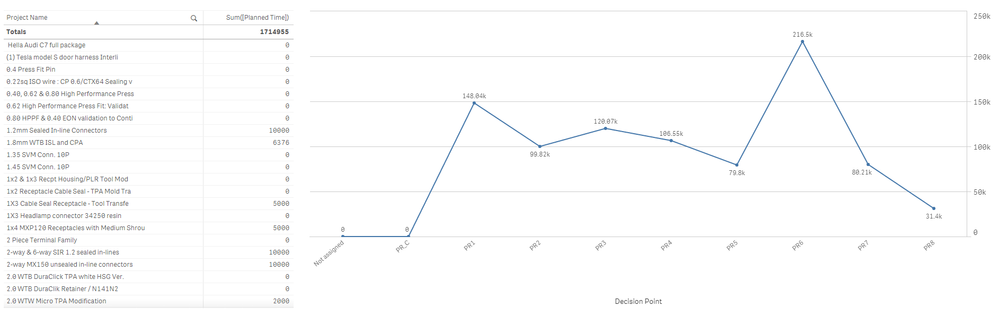# App Development

Announcements
Qlik Insider: SaaS capabilities for Data Integration and Analytics, May 25th! Register Now
cancel
Showing results for
Did you mean:Creator II

## Multiply defined values across a field in Bar Chat in Qlik Sense

Hi Everyone,

If some one could assist me in solving the below logic-

Table 1

 Project Name Planned Time ABC 700 XYZ 154

Table 2

 Phase Percentage P1 16.05% P2 7.80% P3 10.13% P4 11.14% P5 10.11% P6 25.38% P7 10.88% P8 8.32%

Table 3 - Output

 Project Name P1 P2 P3 P4 P5 P6 P7 P8 ABC 112 55 71 78 71 178 76 58 XYZ 23 11 15 16 15 37 16 12

Each Project will be having P1 to P8( as shown in Table 2)

P1 is calculated by = (Planed Time) * (P1 Percentage)

Ex : ABC = 700 * 16.05%

P2 is calculated by = (Planed Time) * (P2 Percentage)

Ex : ABC = 700 * 7.80%

P3 is calculated by = (Planed Time) * (P3 Percentage)

Ex : ABC = 700 * 10.13%

P4 is calculated by = (Planed Time) * (P4 Percentage)

Ex : ABC = 700 * 11.14%

P5 is calculated by = (Planed Time) * (P5 Percentage)

Ex : ABC = 700 * 10.11%

P6 is calculated by = (Planed Time) * (P6 Percentage)

Ex : ABC = 700 * 25.38%

P7 is calculated by = (Planed Time) * (P7 Percentage)

Ex : ABC = 700 * 10.88%

P8 is calculated by = (Planed Time) * (P8 Percentage)

Ex : ABC = 700 * 8.32%

Note : Percentages values are fixed as defined in Table 2.

I want the logic to use in Bar chart( may be in measure ), refer the Image attached.Bar Chart

LP27

Labels (4)

• ### Script Logic

2 RepliesMVP

ThisCreator II
Author

Thanks for that logic,

I actually wanted the tables values in line chart shown in image below.

so if i make selection on Project Names, it should calculate for all PR1 to PR8 in the chart.

I have attached QVF for your understanding.Line Chart